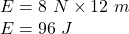## 3. What is the potential energy of a 8 Newton book sitting on a shelf that is 12 meters high?​

Question

3. What is the potential energy of a 8 Newton book sitting on a shelf that is 12 meters high?​

in progress 0
6 months 2021-08-01T17:05:53+00:00 1 Answers 3 views 0

## Answers ( )

P = 96 J

Explanation:

Given that,

Weight of the book, W = mg = 8 N

It is placed at a height of 12 m

We need to find the potential energy of the book. The potential energy of an object is given by the formula as follows :

E = mgh

mg = WeightSo, the potential energy of the book is 96 J.